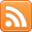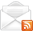# ASP.NET,C#.NET,VB.NET,JQuery,JavaScript,Gridview

aspdotnet-suresh offers C#.net articles and tutorials,csharp dot net,asp.net articles and tutorials,VB.NET Articles,Gridview articles,code examples of asp.net 2.0 /3.5,AJAX,SQL Server Articles,examples of .net technologies

### C# - Get Values from IEnumerable List (String) in C#, VB.NET

Feb 3, 2015
Introduction

Here I will explain how to get values from IEnumerable list or string individually in
c#, vb.net using as.npet. We can get values from IEnumerable list by using First() property or loop through the list to get respective element. IEnumerable list is a base for all collections and its having ability to loop through the collection by using current property, MoveNext and Reset methods in c#, vb.net.

Description:

In previous articles I explained convert datatable to IEnumerable list in c#,
convert datatable to json string in c#, convert datatable to generic list using LINQ, convert datatable to xml string in asp.net, convert datatable or dataset to array in asp.net and many articles relating to asp.net, c#, vb.net, jQuery and JavaScript and. Now I will explain how to get values from IEnumerable list individually in c#, vb.net using

To get values from IEnumerable list individually in c#, vb.net we have different methods

Method 1:

If you want to get first elements from list we need to write the code like as shown below
C# Code

 var data = dt.AsEnumerable().Select(row => new { UserId = row["UserId"].ToString(), UserName = row["UserName"].ToString(), Education = row["Education"].ToString() }); var userid = data.First().UserId; var username = data.First().UserName; var education = data.First().Education;
VB.NET Code

 Dim data = dt.AsEnumerable().[Select](Function(row) New With { _ Key .UserId = row("UserId").ToString(), _ Key .UserName = row("UserName").ToString(), _ Key .Education = row("Education").ToString() _ }) Dim userid = data.First().UserId Dim username = data.First().UserName Dim education = data.First().Education
Method 2:

If you want to get second or third or other elements we need to loop through list

 var data = dt.AsEnumerable().Select(row => new { UserId = row["UserId"].ToString(), UserName = row["UserName"].ToString(), Education = row["Education"].ToString() }).ToList(); string userid, username, education; for (int i = 0; i < data.Count; i++) { userid=data[i].UserId.ToString(); username = data[i].UserName.ToString(); education = data[i].Education.ToString(); }
VB.NET Code

 Dim data = dt.AsEnumerable().[Select](Function(row) New With { _ Key .UserId = row("UserId").ToString(), _ Key .UserName = row("UserName").ToString(), _ Key .Education = row("Education").ToString() _ }).ToList() Dim userid As String, username As String, education As String For i As Integer = 0 To data.Count - 1 userid = data(i).UserId.ToString() username = data(i).UserName.ToString() education = data(i).Education.ToString() Next

 If you enjoyed this post, please support the blog below. It's FREE! Get the latest Asp.net, C#.net, VB.NET, jQuery, Plugins & Code Snippets for FREE by subscribing to our Facebook, Twitter, RSS feed, or by email.Subscribe by RSSSubscribe by Email